# Test: Work & Rate

## 8 Questions MCQ Test Practice Questions for GMAT | Test: Work & Rate

Description
Attempt Test: Work & Rate | 8 questions in 10 minutes | Mock test for GMAT preparation | Free important questions MCQ to study Practice Questions for GMAT for GMAT Exam | Download free PDF with solutions
QUESTION: 1

### Machine A and Machine B can produce 1 widget in 3 hours working together at their respective constant rates. If Machine A's speed were doubled, the two machines could produce 1 widget in 2 hours working together at their respective rates. How many hours does it currently take Machine A to produce 1 widget on its own?

Solution:

Let a be the number of hours it takes Machine A to produce 1 widget on its own. Let b be the number of hours it takes Machine B to produce 1 widget on its own.

The question tells us that Machines A and B together can produce 1 widget in 3 hours. Therefore, in 1 hour, the two machines can produce 1/3 of a widget. In 1 hour, Machine A can produce 1/a widgets and Machine B can produce 1/b widgets. Together in 1 hour, they produce 1/a + 1/b = 1/3 widgets.

If Machine A's speed were doubled it would take the two machines 2 hours to

produce 1 widget. When one doubles the speed, one cuts the amount of time it takes in half. Therefore, the amount of time it would take Machine A to produce 1 widget would be a/2. Under these new conditions, in 1 hour Machine A and B could produce 1/(a/2) + 1/b = 1/2 widgets.  We now have two unknowns and two different equations. We can solve for a.

The two equations:
2/a + 1/b = 1/2 (Remember, 1/(a/2) = 2/a)
1/a + 1/b = 1/3

Subtract the bottom equation from the top:
2/a – 1/a = 1/2 – 1/3
1/a = 3/6 – 2/6
1/a = 1/6
Therefore, a = 6.

QUESTION: 2

### Adam and Brianna plan to install a new tile floor in a classroom. Adam works at a constant rate of 50 tiles per hour, and Brianna works at a constant rate of 55 tiles per hour. If the new floor consists of exactly 1400 tiles, how long will it take Adam and Brianna working together to complete the classroom floor?

Solution:

Because Adam and Brianna are working together, add their individual rates to find their combined rate:

50 + 55 = 105 tiles per hour

The question asks how long it will take them to set 1400 tiles.

Time = Work / Rate = 1400 tiles / (105 tiles / hour) = 40/3 hours = 13 and 1/3 hours = 13 hours and 20 minutes

QUESTION: 3

### A copy machine, working at a constant rate, makes 35 copies per minute. A second copy machine, working at a constant rate, makes 55 copies per minute. Working together at their respective rates, how many copies do the two machines make in half an hour?

Solution:

To find the combined rate of the two machines, add their individual rates:
35 copies/minute + 55 copies/minute = 90 copies/minute.
The question asks how many copies the machines make in half an hour, or 30 minutes.
90 copies/minute × 30 minutes = 2,700 copies.

QUESTION: 4

Tom, working alone, can paint a room in 6 hours. Peter and John, working independently, can paint the same room in 3 hours and 2 hours, respectively. Tom starts painting the room and works on his own for one hour. He is then joined by Peter and they work together for an hour. Finally, John joins them and the three of them work together to finish the room, each one working at his respective rate. What fraction of the whole job was done by Peter?

Solution:

Peter's individual rate is 1 job / 3 hours.
Peter joins Tom and they work together for another hour; Peter and Tom's respective individual rates can be added together to calculate their combined rate: 1/6 + 1/3 = 1/2.
Working together then they will complete 1/2 of the job in the 1 hour they work together.

At this point, 2/3 of the job has been completed (1/6 by Peter alone + 1/2 by Peter and Tom), and 1/3 remains.

When John joins Tom and Peter, the new combined rate for all three is: 1/6 + 1/3 + 1/2 = 1.
The time that it will take them to finish the remaining 1/3 of the job can be solved:
rt = w  →    (1)(t) = 1/3 →       t = 1/3.
The question asks us for the fraction of the job that Peter completed. In the hour that Peter worked with Tom he alone completed: rt = w    →  w = (1/3)(1) = 1/3 of the job.
In the last 1/3 of an hour that all three worked together, Peter alone completed:
(1/3)(1/3) = 1/9 of the job.
Adding these two values together, we get 1/3 + 1/9 of the job = 4/9 of the job.

QUESTION: 5

Machine A can complete a certain job  in X hours. Machine B can complete the same job in y hours. If A and B work together at their respective rates to complete the job, which of the following represents the fraction of the job that B will not have to complete because of A's help?

Solution:

We can solve this problem as a VIC (Variable In Answer Choice) and plug in values for the two variables, x and y. Let's say x = 2 and y = 3.

Machine A can complete one job in 2 hours. Thus, the rate of Machine A is 1/2.

Machine B can complete one job in 3 hours. Thus, the rate of Machine B is 1/3.

The combined rate for Machine A and Machine B working together is: 1/2 + 1/3 = 5/6.

Using the equation (Rate)(Time) = Work, we can plug 5/6 in for the combined rate, plug 1 in for the total work (since they work together to complete 1 job), and calculate the total time as 6/5 hours.

The question asks us what fraction of the job machine B will NOT have to complete because of A's help. In other words we need to know what portion of the job machine A alone completes in that 6/5 hours.

A's rate is 1/2, and it spends 6/5 hours working. By plugging these into the RT=W formula, we calculate that, A completes (1/2)(6/5) = 3/5 of the job. Thus, machine B is saved from having to complete 3/5 of the job.

If we plug our values of x = 2 and y = 3 into the answer choices, we see that only answer choice E yields the correct value of 3/5.

QUESTION: 6

Lindsay can paint 1/x of a certain room in 20 minutes. What fraction of the same room can Joseph paint in 20 minutes if the two of them can paint the room in an hour, working together at their respective rates?

Solution:

We can solve this problem as a VIC (Variable In answer Choice) and plug in values for the variable x. Let’s say x = 6.  (Note that there is a logical restriction here in terms of the value of x. Lindsay has to have a rate of less than less than 1 room per hour if she needs Joseph’s help to finish in an hour).

If Lindsay can paint 1/6 of the room in 20 minutes (1/3 of an hour), her rate is 1/2.
rt = w
r(1/3) = 1/6
r = 1/2

Let J be the number of hours it takes Joseph to paint the entire room. Joseph’s rate then is 1/J.  Joseph and Lindsay’s combined rate is 1/2 + 1/J, which can be simplified:
1/2 + 1/J  →   J / 2J + 2 / 2J     (J + 2) / 2J

If the two of them finish the room in one hour, using the formula of rt = w, we can solve for J.
rt = w and t = 1 (hour), w = 1 (job)

((J + 2) / 2J )(1) = 1    → J + 2 = 2J   → J =2
That means that Joseph’s rate is 1/2, the same as Lindsay’s. The question though asks us what fraction of the room Joseph would complete in 20 minutes, or 1/3 of an hour.
rt = w
(1/2)(1/3) = w
w = 1/6

Now we must look at the answer choices to see which one is equal to 1/6 when we plug in x = 6. Only C works: (6 – 3) / 18 = 1/6.

QUESTION: 7

One smurf and one elf can build a treehouse together in two hours, but the smurf would need the help of two fairies in order to complete the same job in the same amount of time. If one elf and one fairy worked together, it would take them four hours to build the treehouse. Assuming that work rates for smurfs, elves, and fairies remain constant, how many hours would it take one smurf, one elf, and one fairy, working together, to build the treehouse?

Solution:

The combined rate of individuals working together is equal to the sum of all the individual working rates.

Let s = rate of a smurf, e = rate of an elf, and f = rate of a fairy. A rate is expressed in terms of treehouses/hour. So for instance, the first equation below says that a smurf and an elf working together can build 1 treehouse per 2 hours, for a rate of 1/2 treehouse per hour.
s + e = 1/2

2) s + 2 f = 1/2
e + f = 1/4

The three equations can be combined by solving the first one for s in terms of e, and the third equation for f in terms of e, and then by substituting both new equations into the middle equation.

1) s = 1/2 – e
2) (1/2 – e) + 2 (1/4 – e) = 1/2
3) f = 1/4 – e
Now, we simply solve equation 2 for e:
(1/2 – e) + 2 (1/4 – e) = 1/2
2/4 – e + 2/4 – 2 e = 2/4
4/4 – 3e = 2/4
-3e = -2/4
e = 2/12
e = 1/6

Once we know e, we can solve for s and f:

s = 1/2 – e
s = 1/2 – 1/6
s = 3/6 – 1/6
s = 2/6
s = 1/3
f = 1/4 – e
f = 1/4 – 1/6
f = 3/12 – 2/12
f = 1/12
We add up their individual rates to get a combined rate:
e + s + f =
1/6 + 1/3 + 1/12 =
2/12 + 4/12 + 1/12 = 7/12

Remembering that a rate is expressed in terms of treehouses/hour, this indicates that a smurf, an elf, and a fairy, working together, can produce 7 treehouses per 12 hours. Since we want to know the number of hours per treehouse, we must take the reciprocal of the rate. Therefore we conclude that it takes them 12 hours per 7 treehouses, which is equivalent to 12/7 of an hour per treehouse.

QUESTION: 8

paint crew gets a rush order to paint 80 houses in a new development. They paint the first y houses at arate of x houses per week. Realizing that they'll be late at this rate, they bring in some more painters andpaint the rest of the houses at the rate of 1.25x houses per week. The total time it takes them to paint all thehouses under this scenario is what fraction of the time it would have taken if they had painted all the housesat their original rate of x houses per week?

Solution:

Rate is defined as distance divided by time.
Therefore:
The RATE of machine A =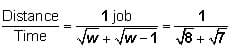The RATE of machine B =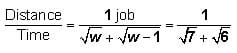The COMBINED RATE of machine A and machine B  =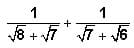This expression can be simplified by eliminating the roots in the denominators as follows: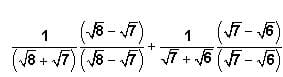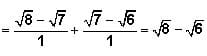The question asks us for the time, t, that it will take both machines working together to finish one job.

Using the combined rate above and a distance of 1 job, we can solve for t as follows: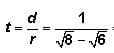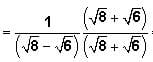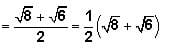The correct answer is choice B.Use Code STAYHOME200 and get INR 200 additional OFF Use Coupon Code